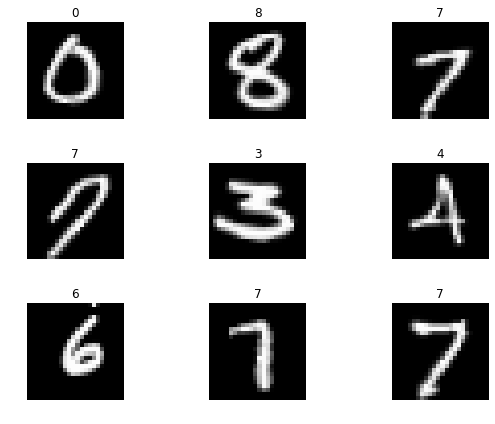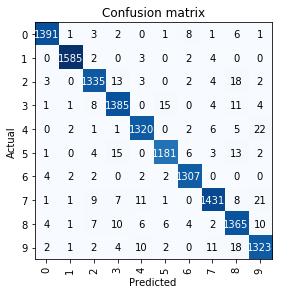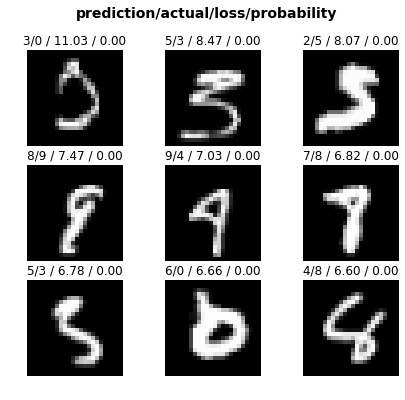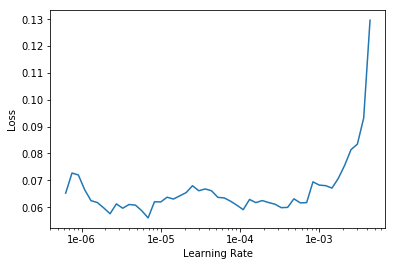After a bad first attempt at creating an image classification model, I trained a model on the MNIST database of handwritten digits this time around. This post is actually a jupyter notebook, which you can find here.

## The Project

``````from fastai.vision import *
``````
``````path = Path('/home/jupyter/projects/data/mnist-handwritten-digits')
``````

There was no need of downloading the images this time - I used images provided at the fastai website from the MNIST database of handwritten digits.

### View Data

``````np.random.seed(2)
data = ImageDataBunch.from_folder(path, train="training", test="testing", valid_pct=0.2, ds_tfms=get_transforms(do_flip=False), size=24).normalize(mnist_stats)
``````
``````data.classes, data.c, len(data.train_ds), len(data.valid_ds)
``````
``````(['0', '1', '2', '3', '4', '5', '6', '7', '8', '9'], 10, 56000, 14000)
``````
``````data.show_batch(rows = 3, figsize=(8,6))
``````### Train Model

``````learn = cnn_learner(data, models.resnet34, metrics=error_rate)
``````
``````learn.fit_one_cycle(4)
``````
epoch train_loss valid_loss error_rate time
0 0.629089 0.367164 0.114357 00:53
1 0.281252 0.141664 0.042786 00:51
2 0.206387 0.094406 0.027857 00:52
3 0.154552 0.086221 0.026929 00:51
``````learn.save('stage-1')
``````

### Interpretation

``````interp = ClassificationInterpretation.from_learner(learn)
``````
``````interp.plot_confusion_matrix()
````````````interp.plot_top_losses(9, figsize=(7, 6), heatmap=False)
``````### Fine Tuning

``````learn.unfreeze()
learn.fit_one_cycle(1)
``````
epoch train_loss valid_loss error_rate time
0 0.056507 0.020995 0.006500 01:02
``````learn.lr_find()
``````
``````LR Finder is complete, type {learner_name}.recorder.plot() to see the graph.
``````
``````learn.recorder.plot()
````````````learn.unfreeze()
learn.fit_one_cycle(2, max_lr=slice(1e-6,1e-4))
``````
epoch train_loss valid_loss error_rate time
0 0.051320 0.019155 0.005857 01:03
1 0.045109 0.017994 0.005571 01:02
``````learn.save('stage-2')
``````